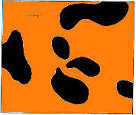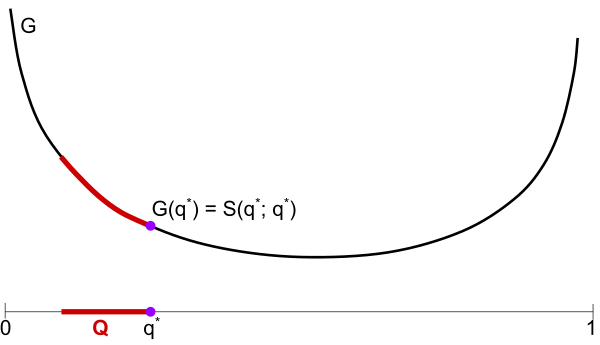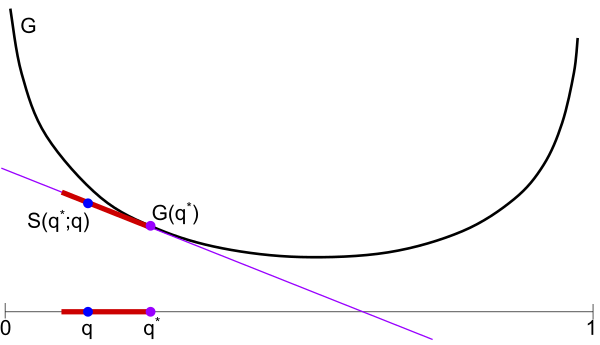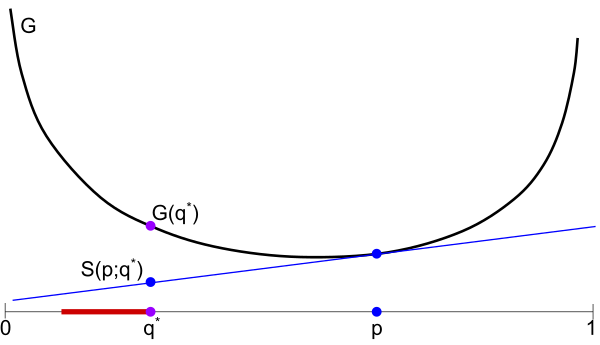# The Tiger's Stripes

A technical blog on math, computer science, and game theory.

Author: Bo Waggoner RSS feed# Risk Aversion and Max-Entropy

Posted: 2016-10-02.

I want to share a nice little problem that came up in discussion between Yiling Chen, Madhu Sudan, and myself. It turns out to have a cute solution that connects the geometry of proper scoring rules with a "max-entropy" rule.

A primer on proper scoring rules will be useful first!

## The Tale of Rita

Once upon a time, Risk-Averse Rita worked as a weather reporter in Incentiveland playing the following game each day:

1. Rita is given a set $Q$ of possible probability distributions over the day's weather outcome, e.g. $Q \subseteq \Delta_{\{\text{rain, cloud, sun}\}}$.
2. Rita makes a prediction $p$, a distribution over the day's weather outcome.
3. Nature picks a true distribution $q$ from $Q$, perhaps adversarially depending on Rita's prediction.
4. The true weather outcome $e$ is drawn according to $q$.
5. Rita is scored with a proper scoring rule $S(p,e)$.

In the case where $|Q| = 1$, i.e. Rita knows the true distribution $q$ of nature, then properness of the scoring rule implies that she should just report $q$. Now, if Rita is risk averse and wishes to maximizes her minimum expected score, how should she play? In math, she wants to solve

$\max_p \min_{q \in Q} \mathbb{E}_{e\sim q} S(p, e) .$ Recalling the notation $S(p;q)$ for expected score of report $p$ when $e \sim q$, this is $\max_p \min_{q \in Q} S(p;q) .$

## The Solution

Luckily, Rita found an optimal strategy. The intuitive statement of the solution is:

If $Q$ is a convex set, then the optimal strategy is to assume the adversary will pick the "max-entropy" distribution in $Q$ and best-respond accordingly.

Furthermore, in this case, the adversary cannot improve on actually picking the "max-entropy" distribution.

Why do I have quotes around "max-entropy"? Because the measure of entropy changes depending on the scoring rule.

If you read my post on generalized entropies, you know that:

1. Every proper scoring rule maps to a convex function $G$ where $G(q)$ is the expected score for optimally (truthfully) reporting given belief $q$.
2. The concave function $F = -G$ can be interpreted as a "generalized entropy" function under an axiomatic approach.
For example, the log scoring rule is $S(p,e) = \log p(e)$, so its expected truthful score function is $\sum_e p(e) \log p(e) = -H(p)$, so its corresponding generalized entropy is just Shannon entropy. For another example, the quadratic scoring rule is $S(p,e) = 2p(e) - \sum_{e'} p(e')^2$ and has corresponding $G(p) = \sum_e p(e)^2$, or generalized entropy $-\|p\|_2^2$, i.e. the collision probability.

Theorem. Let $Q$ be any convex set of distributions and suppose Rita faces scoring rule $S$ with differentiable convex expected score $G$. To solve the problem $\arg\max_p \min_{q \in Q} S(p;q) ,$ Rita's optimal strategy is to report $q^* := \arg\min_{q \in Q} G(Q) .$

Proof. It may be helpful to first see the pictures below. First, we will show that if Rita picks $p=q^*$, then her expected score is at least $G(q^*)$ for any choice of nature (achieved when nature chooses $q^*$). Then, we will show that any other report $p \neq q^*$ results in a worse score.

Claim 1. $\min_{q\in Q} S(q^*;q) = G(q^*)$.

Proof of claim: We just want to show for all $q \in Q$ \begin{align} S(q^*; q) - G(q^*) &\geq 0 \\ \iff ~ \langle dG(q^*), q-q^* \rangle &\geq 0 \end{align} using the scoring rule characterization $S(p;q) = G(p) + \langle dG(p), q-p \rangle$ where $dG(p)$ is the subgradient (i.e. gradient) of $G$ at point $p$.

Now we just want to prove $\langle dG(q^*), q-q^* \rangle \geq 0$ for all $q \in Q$. But since $G$ is differentiable, this is actually equal to the directional derivative of $G$ in the direction $q-q^*$: $\langle dG(q^*), q-q^* \rangle = \lim_{h\to 0} \frac{G(q^* + h(q-q^*)) - G(q^*)}{h} .$ Now, we assumed $Q$ is a convex set, and both $q^*,q \in Q$. So for every $0 \leq h \leq 1$, the point $q' := q^* + h(q-q^*)$ is in $Q$. Furthermore, since we assumed $q^* = \arg\min_{p \in Q} G(p)$, we have $G(q') - G(q) \geq 0$.

So the right side is a limit over nonnegative terms, so the left side cannot be negative.

Claim 2. For all $p$, $\min_{q \in Q} S(p;q) \leq G(q^*)$.

Proof of claim: In particular, $\min_{q \in Q} \leq S(p;q^*)$ because $q^* \in Q$. Now by definition of a proper scoring rule, $S(p;q^*) \leq S(q^*;q^*) = G(q^*)$.

Here we have a binary outcome problem, e.g. the weather is either sunny or not, with probability of sun on the horizontal axis. Plots the convex expected score function $G$ for a scoring rule $S$, and the convex set $Q$ of possible probability distributions of nature.Above: $q^*$ is the "max-entropy" (minimum $G(q)$) among the set $Q$. I claim Rita should pick $q^*$.

Below: Illustration of Claim 1. Supposing Rita reports $q^*$, then nature's best response is $q^*$, as any other choice $q \in Q$ results in a higher expected score $S(q^*;q)$. This shows Rita can achieve $G(q^*)$ by picking $q^*$.Below: Illustration of Claim 2. If Rita makes any other prediction $p \neq q^*$, nature can force her to achieve worse than $G(q^*)$ by picking $q^*$.Below: By flipping $G$, we can view it as a generalized entropy $F$ where $q^*$ is the max-entropy distribution in $Q$.## Questions

Here are some exercises you might enjoy followed by open questions that would be nice to resolve, at least for Rita's sake.

Exercise 1.
Rita's cousin, Risk-Neutral Rick, has a "meta-belief" in the form of a distribution $r$ over $Q$, i.e. $r$ assigns a probability to each $q \in Q$ as being the correct belief. How should Rick report to maximize expected score, i.e. what $p$ solves

$\max_p \mathbb{E}_{q \sim r} \mathbb{E}_{e \sim q} S(p, e) ~ ?$

Exercise 2.
Suppose $Q$ is not a convex set; give a counterexample to the theorem.

Open question 1.
Suppose $Q$ is not a convex set. I would guess that Rita's optimal report is the max-entropy $q$ in the convex hull of $Q$. Prove me right, or disprove it and find Rita's optimal strategy instead.

Open question 2.
What if $G$ is convex, but not differentiable?
The theorem probably still holds, but the proof needs to be much more careful. What happens when $G$ is non-differentiable is that, at some points $q$, there are multiple choices of subgradient $dG(q)$. If we use the wrong one when constructing the scoring rule $S$, then the theorem won't hold; to see this, picture $G$ with a kink at its minimum (such as $G(x) = |x|$) and convince yourself that, if $Q$ contains a ball around the global minimum, then the subgradient chosen at the minimum must be $0$ for the theorem to hold. Maybe elsewhere you don't need to be so careful, and can just use that the subgradients at $p$ form a convex set; I'm not sure.

Open question / challenge 3.
Extend this theorem, as far as possible, to the case of general decision problems rather than just proper scoring rules.

Limits to 1000 characters. HTML does not work but LaTeX does, e.g. $\$$x^y\$$ displays as$x^y\$.